## Polar Coordinates

The polar coordinates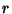and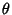are defined by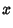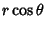(1)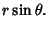(2)

In terms ofand,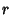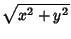(3)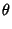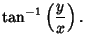(4)

The Arc Length of a polar curve given by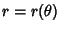is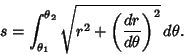(5)

The Line Element is given by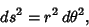(6)

and the Area element by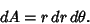(7)

The Area enclosed by a polar curveis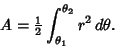(8)

The Slope of a polar functionat the point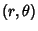is given by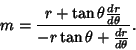(9)

The Angle between the tangent and radial line at the pointis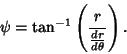(10)

A polar curve is symmetric about the-axis if replacingby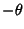in its equation produces an equivalent equation, symmetric about the-axis if replacingby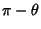in its equation produces an equivalent equation, and symmetric about the origin if replacingby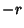in its equation produces an equivalent equation.

In Cartesian coordinates, the Position Vector and its derivatives are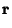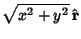(11)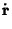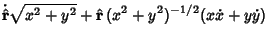(12)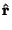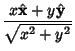(13)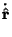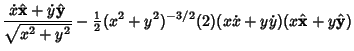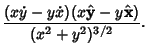(14)

In polar coordinates, the Unit Vectors and their derivatives are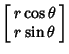(15)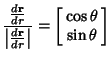(16)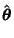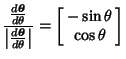(17)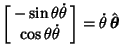(18)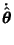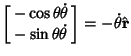(19)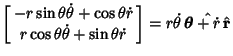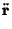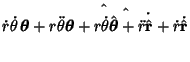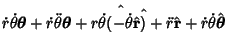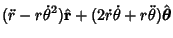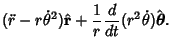(20)

See also Cardioid, Circle, Cissoid, Conchoid, Curvilinear Coordinates, Cylindrical Coordinates, Equiangular Spiral, Lemniscate, Limaçon, Rose